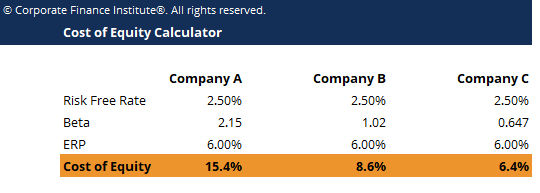# Cost of Equity Calculator

## Cost of Equity Calculator

This cost of equity calculator helps you calculate the cost of equity given the risk free rate, beta and equity risk premium.

This is what the cost of equity calculator looks like:### Cost of Equity Calculator

Cost of Equity is the rate of return a shareholder requires for investing equity into a business. The rate of return an investor requires is based on the level of risk associated with the investment, which is measured as the historical volatility of returns. A firm uses cost of equity to assess the relative attractiveness of investments, including both internal projects and external acquisition opportunities. Companies typically use a combination of equity and debt financing, with equity capital being more expensive.

We can use the CAPM formula to calculate the cost of equity.

E(Ri) = Rf + βi*ERP

Where:

E(Ri) = Expected return on asset i

Rf = Risk free rate of return

βi = Beta of asset i

ERP (Equity Risk Premium) = E(Rm) – Rf

### More Free Templates

For more resources, check out our business templates library to download numerous free Excel modeling, PowerPoint presentation and Word document templates.

• Excel Modeling Templates
• PowerPoint Presentation Templates
• Transaction Document Templates

### Financial Analyst Certification

Become a certified Financial Modeling and Valuation Analyst (FMVA)® by completing CFI’s online financial modeling classes and training program!